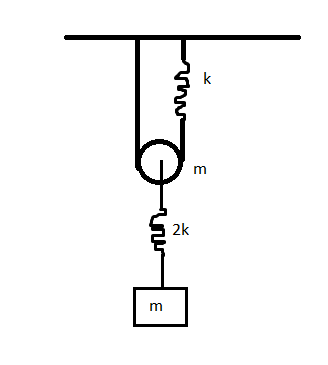# Just hanging around , Medium part2If the time period of SHM of rectangular block can be represented as $T=2\pi \sqrt{\frac{am}{bk}}$. Find ${a^2+b^2}$

Details

• The pulley is smooth with mass $m$, the strings are massless and inextensible.
• The two springs are in phase.
• The pulley system is in a uniform gravitational field.
• Zig-zag lines in fig represents spring of spring constant k and 2k as shown.
• $a$ is of the form $\sqrt c +d$ where $c$ is a square free integer. And $b$ is of the form $e\sqrt f$ where $f$ is square free integer.
• The pulley and the block oscillate in phase with the same frequency

• Try its different variants - Easier and hardest

×

Problem Loading...

Note Loading...

Set Loading...